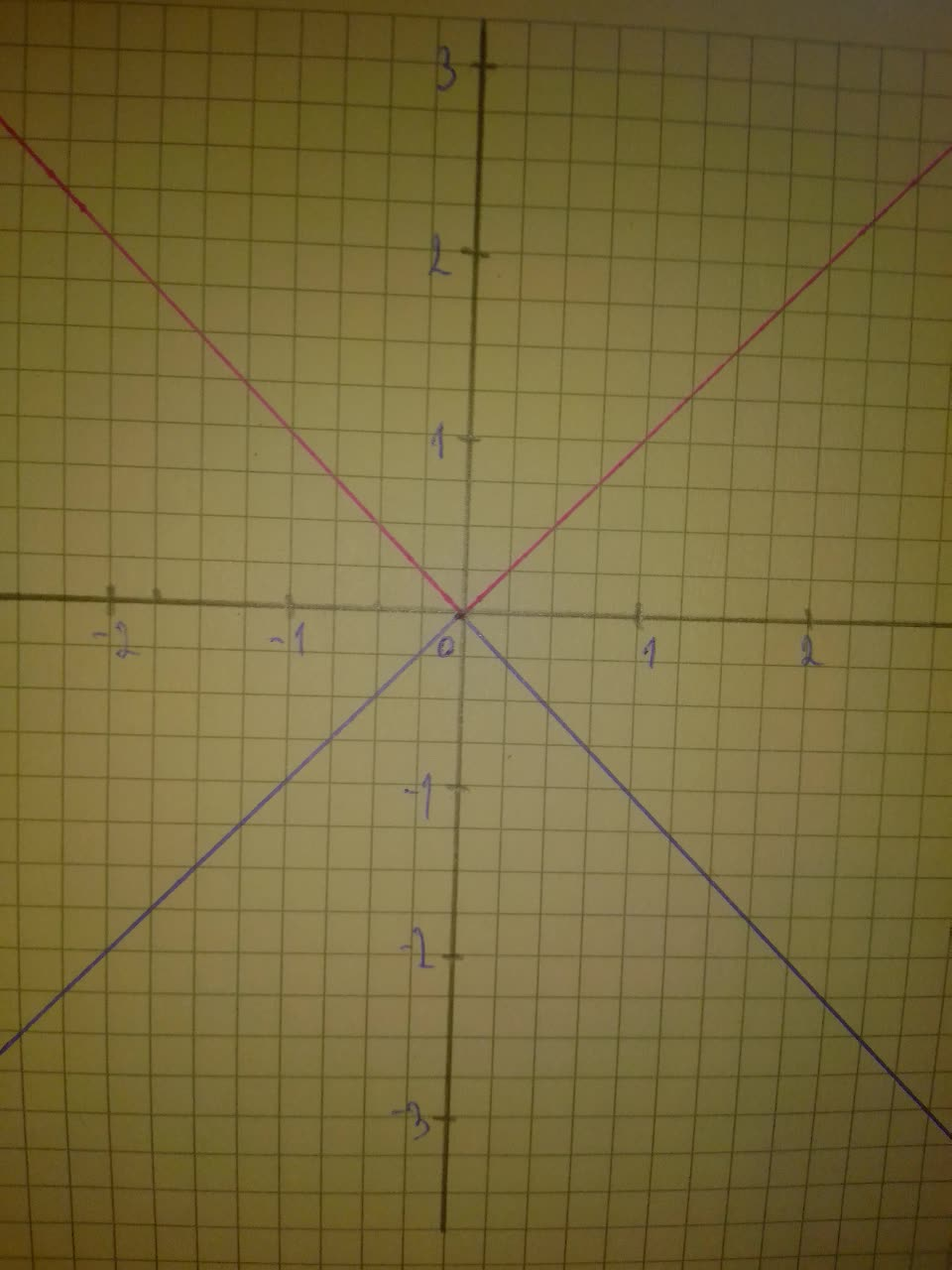Question# Sketch a graph of the function f(x)=-|x|

Exponential models
ANSWEREDSketch a graph of the function. Use transformations of functions whenever possible. $$\displaystyle{f{{\left({x}\right)}}}=-{\left|{x}\right|}$$2021-08-08
Step 1
First graph function $$\displaystyle{y}={\left|{x}\right|}$$
Then, to obtain graph of function $$\displaystyle{f{{\left({x}\right)}}}=-{\left|{x}\right|}$$, do following transformations:
-reflect with respect to x-axis
On graph:
Red - $$\displaystyle{y}={\left|{x}\right|}$$
Blue - $$\displaystyle{f{{\left({x}\right)}}}=-{\left|{x}\right|}$$
Step 2